# A refined brute force method to inform simulation of ordinal response data

Francisco, a researcher from Spain, reached out to me with a challenge. He is interested in exploring various models that estimate correlation across multiple responses to survey questions. This is the context:

• He doesn’t have access to actual data, so to explore analytic methods he needs to simulate responses.
• It would be ideal if the simulated data reflect the properties of real-world responses, some of which can be gleaned from the literature.
• The studies he’s found report only means and standard deviations of the ordinal data, along with the correlation matrices, but not probability distributions of the responses.
• He’s considering simstudy for his simulations, but the function genOrdCat requires a set of probabilities for each response measure; it doesn’t seem like simstudy will be helpful here.

Ultimately, we needed to figure out if we can we use the empirical means and standard deviations to derive probabilities that will yield those same means and standard deviations when the data are simulated. I thought about this for a bit, and came up with a bit of a work-around; the approach seems to work decently and doesn’t require any outrageous assumptions.

I might have kept this between the two of us, but in the process of looking more closely at my solution, I generated a plot that was so beautiful and interesting that I needed to post it. And since I am posting the image, I thought I might as well go ahead and describe the solution in case any one else might find it useful. But first, the plot: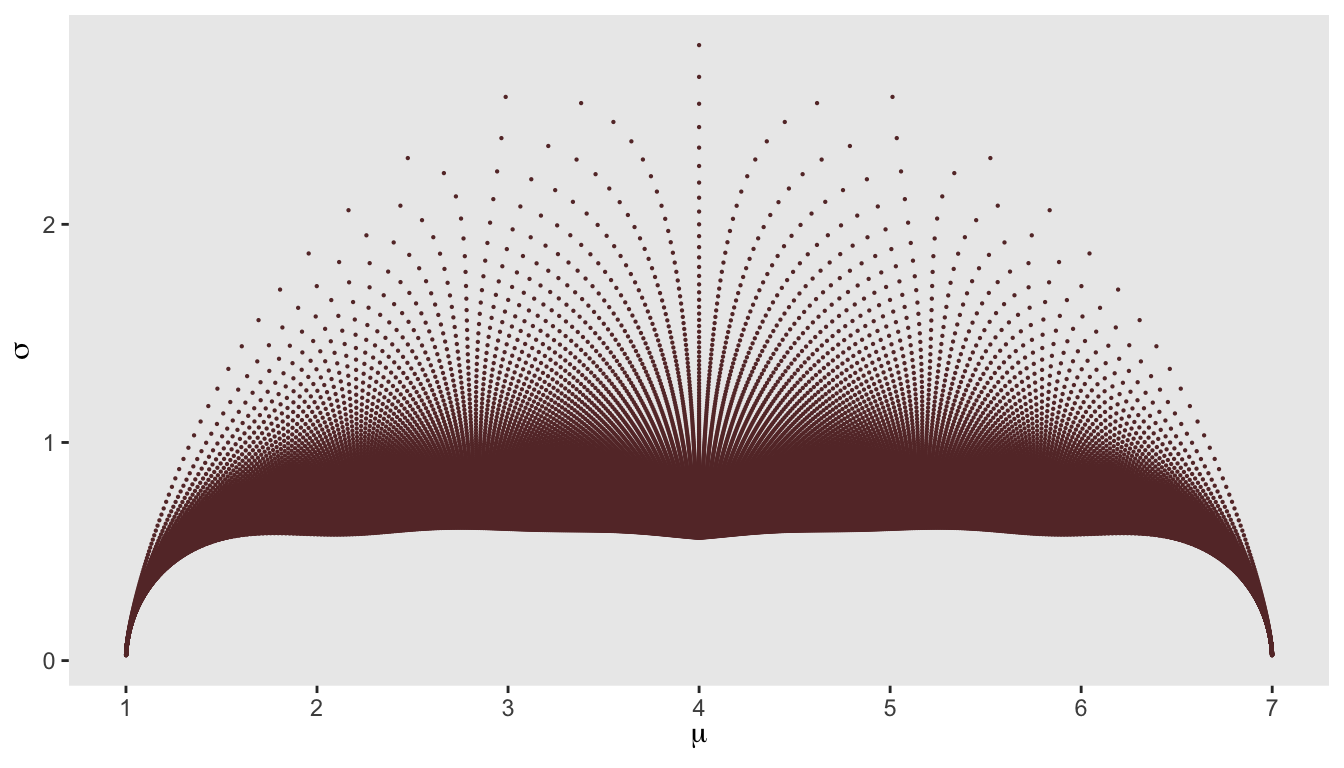### A little more detail

In the simplest scenario, we want to simulate responses from a single survey question with responses ranging from 1 to 7, where 1 might signify totally disagree and 7 would mean totally agree, with gradations in between. Responses collected from a population will be distributed across the seven categories, and the proportion of responses that fall within each category represents the probability of a response.

To inform the simulation, we have a journal article that reports only a mean and standard deviation from responses to that same question collected in an earlier study. The idea is to find the probabilities for the possible responses that correspond to those observed means and standard deviations. That is, how do we go from the mean and standard deviation to a set of probabilities?

The reverse - going from known probabilities to a mean response and standard deviation - is much easier: we just calculate the weighted mean and weighted standard deviations, where the weights are the probabilities.

For example, say the probability distribution of the seven categorical responses is 21%, 20%, 18%, 15%, 13%, 9%, and 4% responding 1, 2, … , and 7, respectively, and represented by this histogram: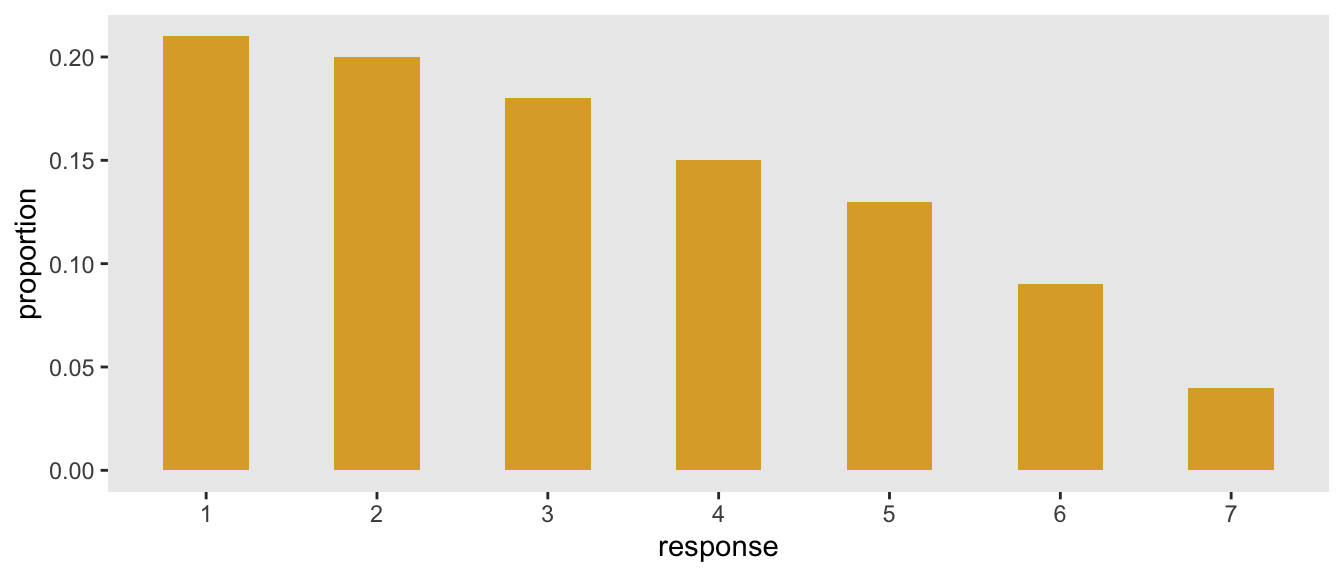Under this distribution the weighted mean and standard deviation are 3.2 and 1.8, respectively:

weighted.mean(x = 1:7, w = c(.21, .20, .18, .15, .13, .09, .04))
##  3.2
weighted.sd(x = 1:7, w = c(.21, .20, .18, .15, .13, .09, .04))
##  1.8

### The brute force approach

My first thought about how use $$\mu$$ and $$\sigma$$ was simple, if a bit crude. Generate a slew of probabilities (like a million or so) and calculate the weighted mean and standard deviation for each distribution. I would look for the probabilities that yielded values that were close to my target (i.e. those that had been reported in the literature).

There are a couple of drawbacks to this approach. First, it is not particularly systematic, since we generating the probabilities randomly, and even though we have large numbers, we are not guaranteed to generate combinations that reflect our targets. Second, there is no reason to think that the generated randomly generated distributions will look like the true distribution. And third, there is no reason to think that, even if we do find a match, the distribution is unique.

I actually went ahead and implemented this approach and found two distributions that also yield $$\mu$$ = 3.2 and and $$\sigma$$ = 1.8 (truth be told, I did this part first and then found the distribution above using the method I will describe in a second):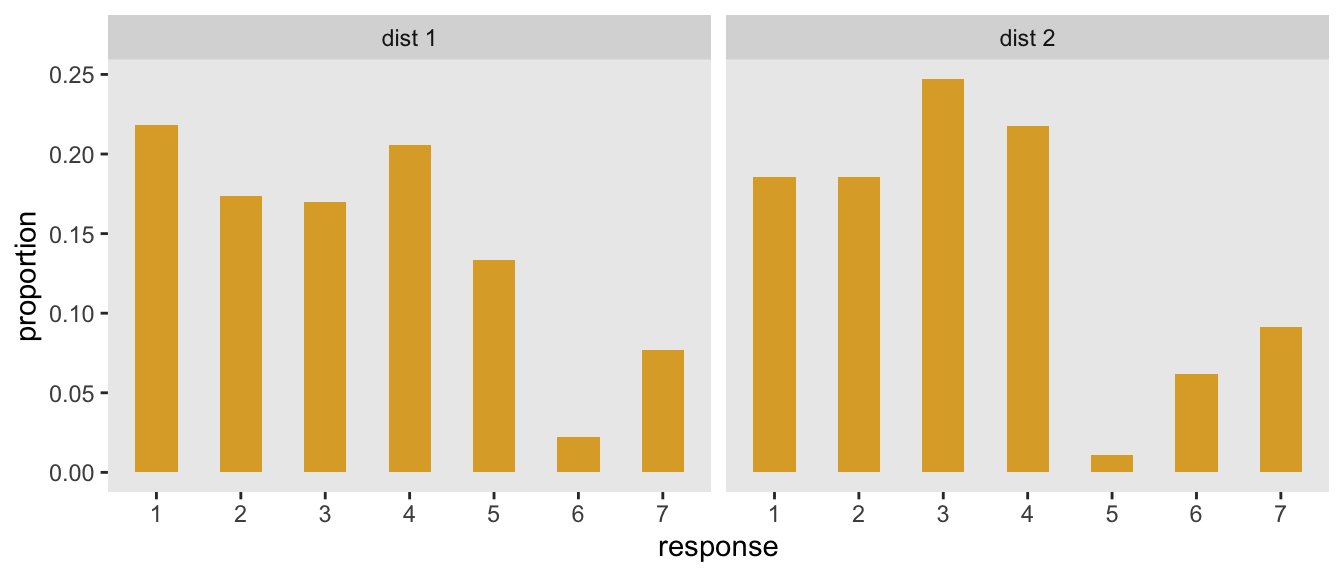Here are the target $$\mu$$’s and $$\sigma$$’s for the distributions on the right and left:

p_left <- c(0.218, 0.174, 0.170, 0.206, 0.134, 0.022, 0.077)
c(weighted.mean(1:7, p_left), weighted.sd(1:7, p_left))
##  3.2 1.8
p_right <- c(0.185, 0.185, 0.247, 0.217, 0.011, 0.062, 0.092)
c(weighted.mean(1:7, p_right), weighted.sd(1:7, p_right))
##  3.2 1.8

### Drawing on the beta distribution

Thinking about probabilities always draws me to the beta family distribution, a continuous distribution from 0 to 1. Theses distributions are parameterized with two shape values, often referred to as $$a$$ and $$b$$. Here are a few probability density functions (pdf’s) for $$(a,b)$$ pairs of (1, 1.6) in yellow, (2, 4) in red, and (2, 2) in blue: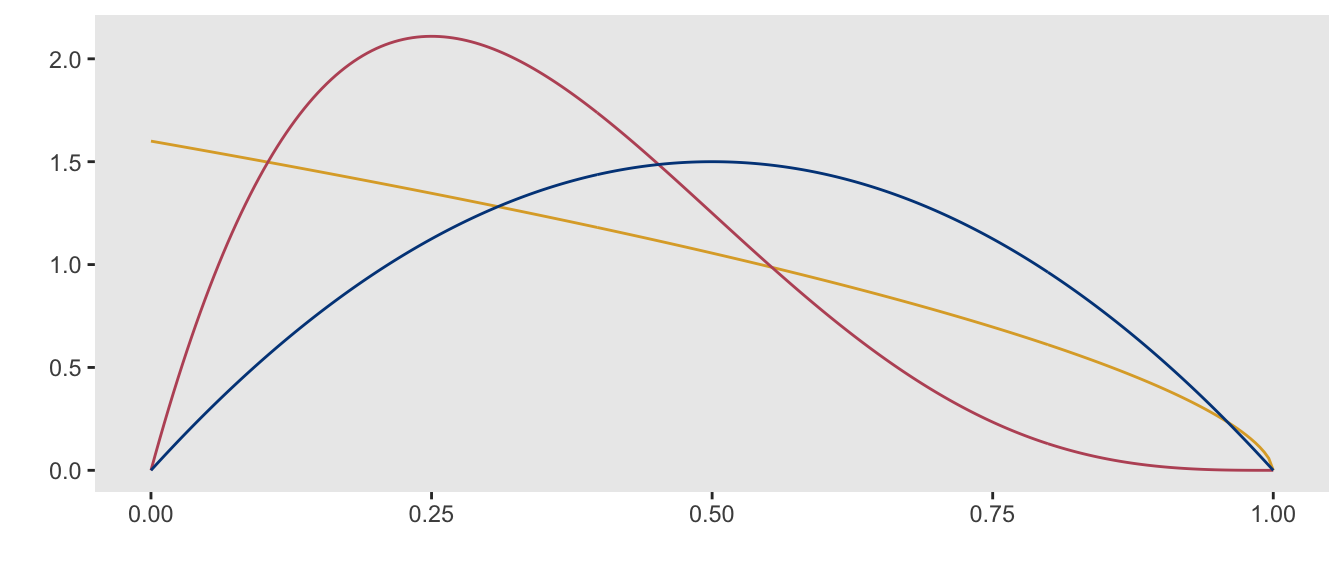I had an idea that generating different pdf’s based on different values of $$a$$ and $$b$$ might provide a more systematic way of generating probabilities. If we carve the pdf into $$K$$ sections (where $$K$$ is the number of responses, in our case 7), then the area under the pdf in the $$k$$th slice could provide the probability for the $$k$$th response. Since each pdf is unique (determined by specific values of $$a$$ and $$b$$), this would ensure different (i.e. unique) sets of probabilities to search through.

Using the example from above where $$a$$ = 1 and $$b$$ = 1.6, here is how the slices look based on the seven categories: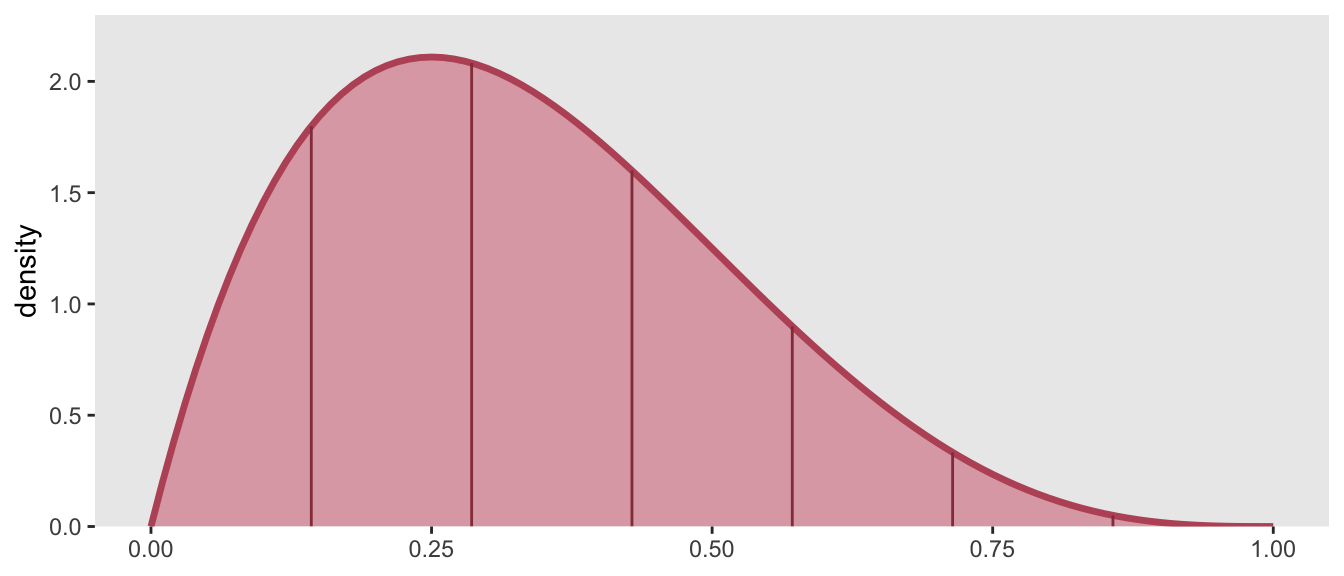The cumulative probability at each slice $$x \in \{1, ..., 7\}$$ is ($$P(X < x/7)$$), and can be calculated in R with the function pbeta:

z <- pbeta((1:7)/7, 2, 4)
z
##  0.15 0.44 0.71 0.89 0.97 1.00 1.00

The probability of for each category is $$P(X = x) = P(X < x) - P(X < (x-1))$$, and is calculated easily:

p <- z - c(0, z[-7])
p
##  0.1518 0.2904 0.2684 0.1786 0.0851 0.0239 0.0018

This is the transformed probability distribution from the continuous beta scale to the discrete categorical scale: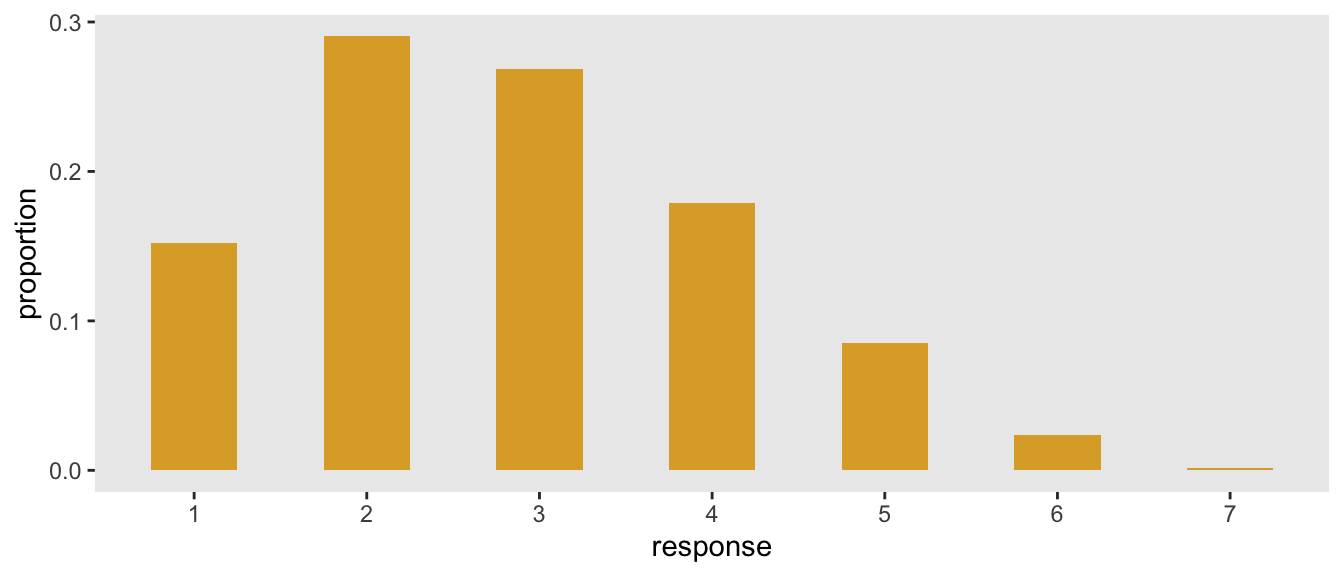And finally here are $$\mu$$ and $$\sigma$$ associated with these values of $$a$$ and $$b$$:

c(weighted.mean(1:7, p), weighted.sd(1:7, p))
##  2.8 1.3

### Brute force, refined

If we create a grid of $$(a, b)$$ values, there will be an associated, and unique, set of probabilities for each pair derived from slicing the pdf into $$K$$ sections And for each of these sets of probabilities, we can calculate the means and standard deviations. We then find the $$(\mu, \sigma)$$ pair that is closest to our target. While this idea is not that much better than the brute force approach suggested above, at least it is now systematic. If we do it in two steps, first by searching for the general region and then zooming in to find a specific set of probabilities, we can really speed things up and use less memory.

Is limiting the search to beta-based distributions justifiable? It might depend on the nature of responses in a particular case, but it does seem reasonable; most importantly, it assures fairly well-behaved distributions that could plausibly reflect a wide range of response patterns. Barring any additional information about the distributions, then, I would have no qualms using this approach. (If it turns out that this is a common enough problem, I would even consider implementing the algorithm as a simstudy function.)

Now, it is time to reveal the secret of the plot (if you haven’t figured it out already). Each point is just the $$(\mu, \sigma)$$ pair generated by a specific $$(a, b)$$ pair. Here is the code, implementing the described algorithm:

get_abp <- function(a, b, size) {

x <- 1:size

z <- pbeta((1:size)/size, a, b)
p <- z - c(0, z[-size])

sigma <- weighted.sd(x, p)
mu <- weighted.mean(x, p)

data.table(a, b, mu, sigma, t(p))
}

get_p <- function(a, b, n) {
ab <- asplit(expand.grid(a = a, b = b), 1)
rbindlist(lapply(ab, function(x) get_abp(x, x,  n)))
}

a <- seq(.1, 25, .1)
b <- seq(.1, 25, .1)

ab_res <- get_p(a, b, 7)

We can fill in the plot with more points by increasing the range of $$a$$ and $$b$$ that we search, but creating such a huge table of look-up values is time consuming and starts to eat up memory. In any case, there is no need, because we will refine the search by zooming in on the area closest to our target.

Here is the plot again, based on $$a$$’s and $$b$$’s ranging from 0.1 to 25, with the superimposed target pair $$(\mu =3.2, \sigma = 1.8)$$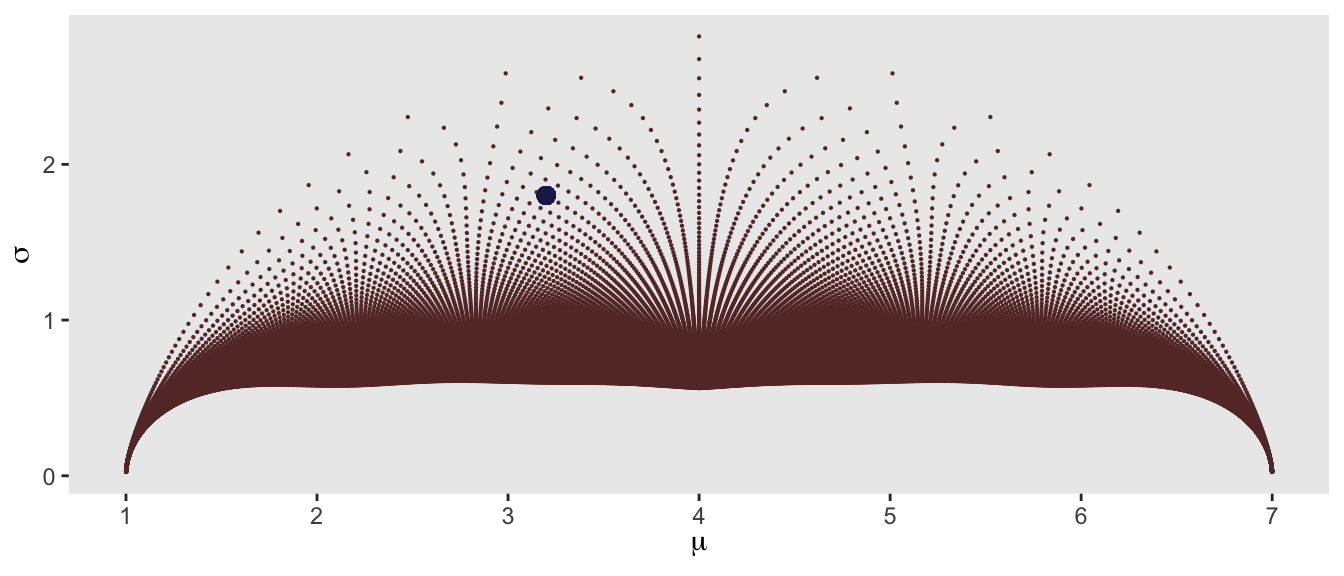To zoom in, we first find the point in the grid that is closest to our target (based on Euclidean distance). We then define a finer grid around this point in the grid, and re-search for the closest point. We do have to be careful that we do not search for invalid values of $$a$$ and $$b$$ (i.e. $$a \le 0$$ and $$b \le 0$$). Once we find our point, we have the associated probabilities.:

t_mu = 3.2
t_s = 1.8

ab_res[, distance := sqrt((mu - t_mu)^2 + (sigma - t_s)^2)]
close_point <- ab_res[distance == min(distance), .(a, b, distance)]

a_zoom<- with(close_point, seq(a - .25, a + .25, length = 75))
b_zoom<- with(close_point, seq(b - .25, b + .25, length = 75))

a_zoom <- a_zoom[a_zoom > 0]
b_zoom <- b_zoom[b_zoom > 0]

res_zoom <- get_p(a_zoom, b_zoom, 7)

Here is the new search region: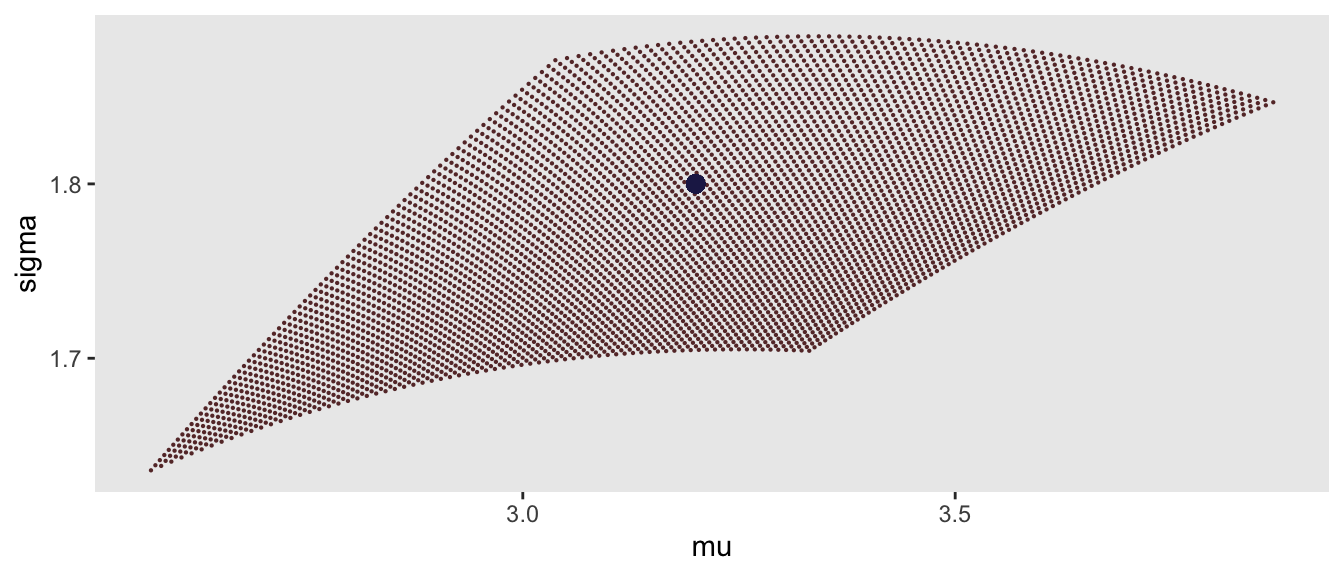And the selection of the point:

res_zoom[, distance := sqrt((mu - t_mu)^2 + (sigma - t_s)^2)]
res_zoom[distance == min(distance)]
##       a   b  mu sigma   V1  V2   V3   V4   V5   V6    V7 distance
## 1: 0.97 1.6 3.2   1.8 0.22 0.2 0.17 0.15 0.12 0.09 0.046   0.0021

### Applying the beta-search to a bigger problem

To conclude, I’ll finish with Francisco’s more ambitious goal of simulating correlated responses to multiple questions. In this case, we will assume four questions, all with responses ranging from 1 to 7. The target ($$\mu, \sigma$$) pairs taken from the (hypothetical) journal article are:

targets <- list(c(2.4, 0.8), c(4.1, 1.2), c(3.4, 1.5), c(5.8, 0.8))

The correlation matrix taken from this same article is:

corMat <- matrix(c(
1.00, 0.09, 0.11, 0.05,
0.09, 1.00, 0.35, 0.16,
0.11, 0.35, 1.00, 0.13,
0.05, 0.16, 0.13, 1.00), nrow=4,ncol=4)

The get_target_prob function implements the search algorithm described above:

get_target_prob <- function(t_mu, t_s, ab_res) {

ab_res[, distance := sqrt((mu - t_mu)^2 + (sigma - t_s)^2)]
close_point <- ab_res[distance == min(distance), .(a, b, distance)]

a_zoom<- with(close_point, seq(a - .25, a + .25, length = 75))
b_zoom<- with(close_point, seq(b - .25, b + .25, length = 75))

a_zoom <- a_zoom[a_zoom > 0]
b_zoom <- b_zoom[b_zoom > 0]

res_zoom <- get_p(a_zoom, b_zoom, 7)

res_zoom[, distance := sqrt((mu - t_mu)^2 + (sigma - t_s)^2)]
baseprobs <- as.vector(res_zoom[distance == min(distance), paste0("V", 1:7)], "double")

baseprobs
}

Calling the function conducts the search and provides probabilities for each question:

probs <- lapply(targets, function(x) get_target_prob(x, x, ab_res))
(probs <- do.call(rbind, probs))
##         [,1]    [,2]   [,3]  [,4]   [,5]    [,6]    [,7]
## [1,] 1.1e-01 4.8e-01 0.3334 0.077 0.0059 8.8e-05 3.7e-08
## [2,] 7.9e-03 8.5e-02 0.2232 0.306 0.2537 1.1e-01 1.3e-02
## [3,] 1.0e-01 2.1e-01 0.2345 0.209 0.1502 7.9e-02 1.7e-02
## [4,] 2.5e-08 5.4e-05 0.0036 0.051 0.2638 5.0e-01 1.8e-01

At least in theory, Francisco can now conduct his simulation study. In this case, I am generating a huge sample size to minimize sampling variation with the hope that we can recover the means, standard deviations and correlations, which, of course, we do:

d_ind <- genData(100000)
dx <- genOrdCat(d_ind, adjVar = NULL, baseprobs = probs, catVar = "y",
corMatrix = corMat, asFactor = FALSE)
apply(as.matrix(dx[, -1]), 2, mean)
## grp1 grp2 grp3 grp4
##  2.4  4.1  3.4  5.8
apply(as.matrix(dx[, -1]), 2, sd)
## grp1 grp2 grp3 grp4
##  0.8  1.2  1.5  0.8
cor(as.matrix(dx[, -1]))
##       grp1 grp2  grp3  grp4
## grp1 1.000 0.08 0.099 0.043
## grp2 0.080 1.00 0.331 0.142
## grp3 0.099 0.33 1.000 0.110
## grp4 0.043 0.14 0.110 1.000

In the end, Francisco seemed to be satisfied with the solution - at least satisfied enough to go to the trouble to have a bottle of wine sent to me in New York City, which was definitely above and beyond. While my wife and I will certainly enjoy the wine - and look forward to being able to travel again so maybe we can enjoy a glass in person - seeing that image emerge from a beta-distribution was really all I needed. Salud.